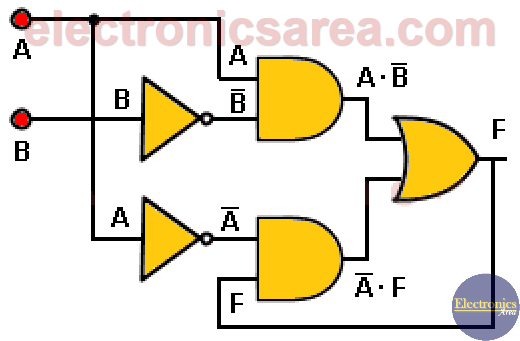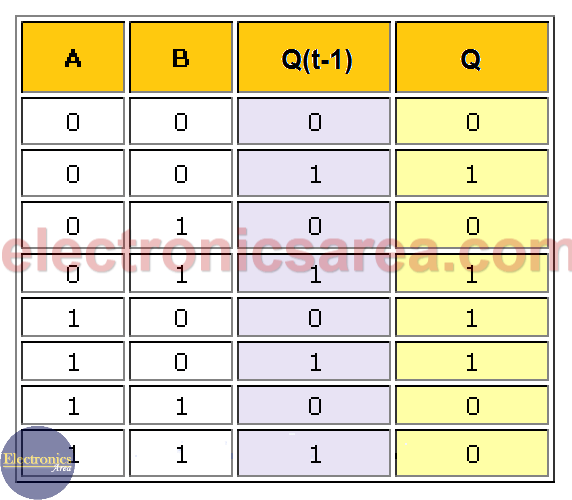# Sequential Circuits Using Logic Gates

The main difference between combinational circuits and sequential circuits is that in sequential circuits there is one or more output signals fed back to one or more inputs to obtain a new output. See the diagram.

It can be seen that the output of the OR gate is fed back and used as an input of the lower AND gate. This means that the Q output of this digital circuit will depend on the inputs (A and B), but it will also depend on the output Q(t-1) (the feedback output) that occurred, an instant before.

In other words, the output Q depends on the inputs A and B and the value that the output had previously Q(t-1))The truth table is shown in the following diagram, where the output Q depends on the present value of the input signals (A, B) as well as the sequence of a past output Q(t-1)).This diagram and its respective truth table are a specific example. Other diagrams can have more inputs A, B, C, … etc), more outputs (Q1, Q2, Q3, …, etc) and more feedback outputs Q1(t-1), Q2(t-1), Q3(t-1), …, etc.) .

In case of having more outputs, it would be necessary to obtain a truth table for each Q output according to the inputs and all the previous outputs that are fed back.

Notes:

• It is important to mention that although the current Q output column shows “0” and “1” for all combinations of A and B, this is not necessarily true.
• The outputs in other sequential circuits can be anywhere within the circuit diagram.
• 1
•
•
•
•
•
•
•
•
1
Share
•
1
Share
• 1
•
•
•
•
•
•
•